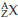×
Get Full Access to Introductory Chemistry - 5 Edition - Chapter 4 - Problem 96p
Get Full Access to Introductory Chemistry - 5 Edition - Chapter 4 - Problem 96p

×

# Write the symbol for each isotope in the form .(a) U-235(b) V-52(c) P-32(d) Xe-144ISBN: 9780321910295 34

## Solution for problem 96P Chapter 4

Introductory Chemistry | 5th Edition

• Textbook Solutions
• 2901 Step-by-step solutions solved by professors and subject experts
• Get 24/7 help from StudySoup virtual teaching assistantsIntroductory Chemistry | 5th Edition

4 5 1 242 Reviews
10
5
Problem 96P

Write the symbol for each isotope in the form.

(a) $$U-235$$

(b) $$V-52$$

(c) $$P-32$$

(d) $$Xe-144$$

Equation Transcription:Text Transcription:

U-235

V-52

P-32

Xe-144

Step-by-Step Solution:

Step 1 of 5

The symbol of an element can be written in an informative way by representing the atomic number as the subscript of the element and atomic mass of the element as the superscript of the symbol.

Step 2 of 5

Step 3 of 5

## Discover and learn what students are asking

Calculus: Early Transcendental Functions : Space Coordinates and Vectors in Space
?In Exercises 1-4, plot the points in the same three-dimensional coordinate system. (a) (0, 4, -5) (b) (4, 0, 5

Unlock Textbook Solution